# Geometry Set 1 2019-2020 SSC (English Medium) 10th Standard Board Exam Question Paper Solution

Geometry [Set 1]
Date & Time: 14th March 2020, 11:00 am
Duration: 2h
1. All questions are compulsory.
2. Use of calculator is not allowed.
3. The numbers to the right of the questions indicate full marks.
4. In the case of MCQ’s Q. No. 1(A) only the first attempt will be evaluated and will be given credit.
5. For every MCQ, the correct alternative (A), (B), (C) or (D) in front of subquestion number is to be written as an answer.

 1
 1.A | For every subquestion 4 alternative answers are given. Choose the correct answer and write the alphabet of it:
 1.A.i

Some question and their alternative answer are given. Select the correct alternative.

Out of the following which is the Pythagorean triplet?

(1, 5, 10)

(3, 4, 5)

(2, 2, 2)

(5, 5, 2)

Concept: Apollonius Theorem
Chapter: [0.02] Pythagoras Theorem
 1.A.ii

Two circles of radii 5.5 cm and 3.3 cm respectively touch each other externally. What is the distance between their centres?

4.4 cm

2.2 cm

8.8 cm

8.9 cm

Concept: Touching Circles
Chapter: [0.03] Circle
 1.A.iii

Fill in the blank using correct alternative.

Distance of point (–3, 4) from the origin is ___________

7

1

5

−5

Concept: Slope of a Line
Chapter: [0.04] Co-ordinate Geometry
 1.A.iv

Find the volume of a cube of side 3 cm:

27 cm3

9 cm3

81 cm3

3 cm3

Concept: Volume of a Combination of Solids
Chapter: [0.07] Mensuration
 1.B | Solve the following questions:
 1.B.i

The ratio of corresponding sides of similar triangles is 3 : 5, then find the ratio of their areas.

Concept: Areas of Two Similar Triangles
Chapter: [0.01] Similarity
 1.B.ii

Find the diagonal of a square whose side is 10 cm.

Concept: Circumference of a Circle
Chapter: [0.07] Mensuration [0.07] Mensuration
 1.B.iii

squareABCD is cyclic. If ∠B = 110°, then find measure of ∠D.

Concept: Converse: If a pair of opposite angles of a quadrilateral is supplementary, then the quadrilateral is cyclic.
Chapter: [0.03] Circle
 1.B.iv

Find the slope of the lines passing through the given point.

A(2, 3), B(4, 7)

Concept: Slope of a Line
Chapter: [0.04] Co-ordinate Geometry
 2
 2.A | Complete and write any TWO activities from the following:
 2.A.i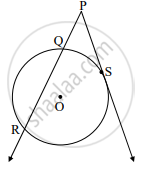In the figure given above, ‘O’ is the centre of the circle, seg PS is a tangent segment and S is the point of contact. Line PR is a secant.
If PQ = 3.6, QR = 6.4, find PS.

Solution:

PS2 = PQ × square ......(tangent secant segments theorem)

= PQ × (PQ + square)

= 3.6 × (3.6 + 6.4)

= 3.6 × square

= 36

∴ PS = square .....(by taking square roots)

Concept: Tangent - Secant Theorem
Chapter: [0.03] Circle
 2.A.ii

If sec θ = 25/7, find the value of tan θ.

Solution:

1 + tan2 θ = sec2 θ

∴ 1 + tan2 θ = (25/7)^square

∴ tan2 θ = 625/49 - square

= (625 - 49)/49

= square/49

∴ tan θ = square/7 ........(by taking square roots)

Concept: Trigonometric Identities
Chapter: [0.06] Trigonometry
 2.A.iii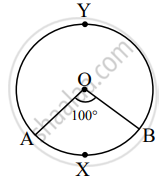In the figure given above, O is the centre of the circle. Using given information complete the following table:

 Type of arc Name of the arc Measure of the arc Minor arc square square Major arc square square
Concept: Property of Sum of Measures of Arcs
Chapter: [0.03] Circle
 2.B | Solve any FOUR subquestions from the following:
 2.B.i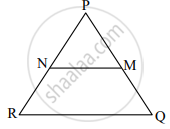In ΔPQR, NM || RQ. If PM = 15, MQ = 10, NR = 8, then find PN.

Concept: Basic Proportionality Theorem Or Thales Theorem
Chapter: [0.01] Similarity
 2.B.ii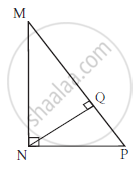In ΔMNP, ∠MNP = 90˚, seg NQ ⊥ seg MP, MQ = 9, QP = 4, find NQ.

Concept: Right-angled Triangles and Pythagoras Property
Chapter: [0.02] Pythagoras Theorem
 2.B.iii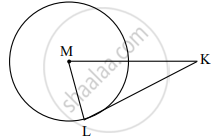In the given figure, M is the centre of the circle and seg KL is a tangent segment. L is a point of contact. If MK = 12, KL = 6sqrt3, then find the radius of the circle.

Concept: Tangent Segment Theorem
Chapter: [0.03] Circle
 2.B.iv

Find the coordinates of midpoint of the segment joining the points (22, 20) and (0, 16).

Concept: The Mid-point of a Line Segment (Mid-point Formula)
Chapter: [0.04] Co-ordinate Geometry
 2.B.v

A person is standing at a distance of 80 m from a church looking at its top. The angle of elevation is of 45°. Find the height of the church.

Concept: Heights and Distances
Chapter: [0.06] Trigonometry
 3
 3.A | Complete and write any ONE activity from the following:
 3.A.i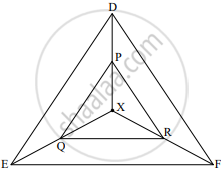In the given figure, X is any point in the interior of the triangle. Point X is joined to the vertices of triangle. seg PQ || seg DE, seg QR || seg EF. Complete the activity and prove that seg PR || seg DF.

Proof:

In ΔXDE, PQ || DE …(Given)

∴ "XP"/"PD" = square/"QE" …(Basic proportionality theorem)…(i)

In ΔXEF, QR || EF …(Given)

∴ "XQ"/square = "XR"/square ..........(square)....(ii)

∴ "XP"/"PD" = square/square ..…[From (i) and (ii)]

∴ seg PR || seg DF …(By converse of basic proportionality theorem

Concept: Converse of Basic Proportionality Theorem
Chapter: [0.01] Similarity
 3.A.ii

If A(6, 1), B(8, 2), C(9, 4) and D(7, 3) are the vertices of squareABCD, show that squareABCD is a parallelogram.

Solution:

Slope of line = ("y"_2 - "y"_1)/("x"_2 - "x"_1)

∴ Slope of line AB = (2 - 1)/(8 - 6) = square .......(i)

∴ Slope of line BC = (4 - 2)/(9 - 8) = square .....(ii)

∴ Slope of line CD = (3 - 4)/(7 - 9) = square .....(iii)

∴ Slope of line DA = (3 - 1)/(7 - 6) = square .....(iv)

∴ Slope of line AB = square ......…[From (i) and (iii)]

∴ line AB || line CD

∴ Slope of line BC = square …[From (ii) and (iv)]

∴ line BC || line DA

Both the pairs of opposite sides of the quadrilateral are parallel.

∴ squareABCD is a parallelogram.

Concept: Slope of a Line
Chapter: [0.04] Co-ordinate Geometry
 3.B |  Solve any TWO subquestions from the following:
 3.B.i

In ∆PQR, point S is the midpoint of side QR. If PQ = 11, PR = 17, PS = 13, find QR.

Concept: Right-angled Triangles and Pythagoras Property
Chapter: [0.02] Pythagoras Theorem
 3.B.ii

Prove the following theorems: Tangent segments drawn from an external point to the circle are congruent

Concept: Tangent Segment Theorem
Chapter: [0.03] Circle
 3.B.iii

Draw a circle with radius 4.1 cm. Construct tangents to the circle from a point at a distance 7.3 cm from the centre.

Concept: Construction of Tangents to a Circle
Chapter: [0.05] Geometric Constructions
 3.B.iv

A metal cuboid of measures 16 cm × 11 cm × 10 cm was melted to make coins. How many coins were made, if the thickness and diameter of each coin was 2 mm and 2 cm respectively? (π = 3.14)

Concept: Concept of Surface Area, Volume, and Capacity
Chapter: [0.07] Mensuration
 4 | Solve any TWO subquestions from the following:
 4.A

In ΔABC, PQ is a line segment intersecting AB at P and AC at Q such that seg PQ || seg BC. If PQ divides ΔABC into two equal parts having equal areas, find "BP"/"AB".

Concept: Areas of Similar Triangles
Chapter: [0.01] Similarity
 4.B

Draw a circle of radius 2.7 cm and draw a chord PQ of length 4.5 cm. Draw tangents at points P and Q without using centre.

Concept: Tangent to a Circle
Chapter: [0.03] Circle
 4.C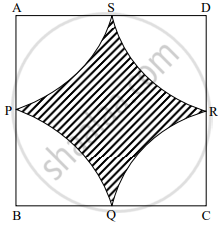In the given figure squareABCD is a square of side 50 m. Points P, Q, R, S are midpoints of side AB, side BC, side CD, side AD respectively. Find area of shaded region

Concept: Surface Area of a Combination of Solids
Chapter: [0.07] Mensuration
 5 | Solve any ONE subquestions from the following:
 5.A

Circles with centres A, B and C touch each other externally. If AB = 3 cm, BC = 3 cm, CA = 4 cm, then find the radii of each circle.

Concept: Concept of Circle - Centre, Radius, Diameter, Arc, Sector, Chord, Segment, Semicircle, Circumference, Interior and Exterior, Concentric Circles
Chapter: [0.03] Circle
 5.B

If sin θ + sin2 θ = 1 show that: cos2 θ + cos4 θ = 1

Concept: Trigonometric Identities
Chapter: [0.06] Trigonometry

#### Request Question Paper

If you dont find a question paper, kindly write to us

View All Requests

#### Submit Question Paper

Help us maintain new question papers on Shaalaa.com, so we can continue to help students

only jpg, png and pdf files

## Maharashtra State Board previous year question papers 10th Standard Board Exam Geometry with solutions 2019 - 2020

Maharashtra State Board 10th Standard Board Exam Geometry question paper solution is key to score more marks in final exams. Students who have used our past year paper solution have significantly improved in speed and boosted their confidence to solve any question in the examination. Our Maharashtra State Board 10th Standard Board Exam Geometry question paper 2020 serve as a catalyst to prepare for your Geometry board examination.
Previous year Question paper for Maharashtra State Board 10th Standard Board Exam Geometry-2020 is solved by experts. Solved question papers gives you the chance to check yourself after your mock test.
By referring the question paper Solutions for Geometry, you can scale your preparation level and work on your weak areas. It will also help the candidates in developing the time-management skills. Practice makes perfect, and there is no better way to practice than to attempt previous year question paper solutions of Maharashtra State Board 10th Standard Board Exam.

How Maharashtra State Board 10th Standard Board Exam Question Paper solutions Help Students ?
• Question paper solutions for Geometry will helps students to prepare for exam.
• Question paper with answer will boost students confidence in exam time and also give you an idea About the important questions and topics to be prepared for the board exam.
• For finding solution of question papers no need to refer so multiple sources like textbook or guides.